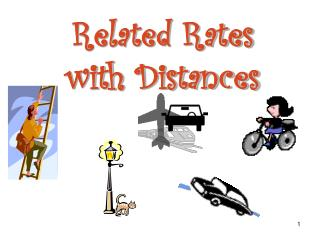Download PresentationRelated Rates with Distances

# Related Rates with Distances - PowerPoint PPT PresentationDownload Presentation## Related Rates with Distances

- - - - - - - - - - - - - - - - - - - - - - - - - - - E N D - - - - - - - - - - - - - - - - - - - - - - - - - - -
##### Presentation Transcript

1. Related Rateswith Distances

2. x y x + y EXAMPLE 1: A man 6 ft. tall walks at a rate of 2 ft./sec away from a lamp post that is 13 ft. high. At what rate is the length of his shadow changing when he is 25 ft. away from the lamp post. xrepresents his distance away from the lamp post. yrepresents the length of his shadow. 13 We want 6 We know What is the relationship betweenx and y? Use similar triangles.

3. 13 x + y 6 y EXAMPLE 1: A man 6 ft. tall walks at a rate of 2 ft./sec away from a lamp post that is 13 ft. high. At what rate is the length of his shadow changing when he is 25 ft. away from the lamp post. A man 6 ft. tall walks at a rate of 2 ft./sec aay from a lamp post that is 13 ft. high. At what rate is the length of his shadow changing when he is 25 ft. away from the lamp post. Use similar triangles. The 25 did not matter.

4. continued EXAMPLE 2: A spotlight on the ground shines on a wall 10 m away. A man 2 m tall walks from the spotlight toward the wall at a speed of 1.2m/s. 10 m

5. How fast is his shadow on the wall decreasing when he is 3 m from the spotlight? Use Similar Triangles y 2 m x 10 Differentiate with respect to t

6. continued EXAMPLE 3: A rocket rising vertically is being tracked by a radar command post on the ground 10 km from the launch pad. How fast is the rocket rising when it is 8 km high and its distance from the radar station is increasing at a rate of 1000 km/h? 10 km

7. z x 10 km continued At any given time From the Pythagorean theorem x 2 + 102 = z2 Differentiate implicitly Find the distance the rocket is from the station when it is 8 km high

8. Substitute into the derivative The rocket is rising at 1600 km/h when it is 8 km high.

9. Example 4: A passenger car approaches a railway crossing from the east at 50 km/h, while a locomotive approaches the crossing from the north at 110 km/h. How fast is the distance between them changing at the moment when the car is 30 km and the train is 40 km from the intersection? At any given time x2 + y2 = z2 Differentiate with respect to t z x y

10. Find the distance between them when the car is 30 m and the train is 40 m from the crossing. 40 m 30 m 50 m Substitute into the derivative The distance between them is changing at 118 km/h

11. 5 m 5 m xm xm y m y m EXAMPLE 5: A 5 m ladder which is leaning against a wall begins to slip. If the top slips down the wall at a rate of 0.60 m/s, how fast is the bottom moving when it is 3 m from the wall?

12. 5 m x m y m continued EXAMPLE 5: A 5 m ladder which is leaning against a wall begins to slip. If the top slips down the wall at a rate of 0.6 m/s, how fast is the bottom moving when it is 3 m from the wall? From the Pythagorean theorem x 2 + y 2 = 25 Differentiate implicitly How fast is the bottom moving?

13. Find the height of the ladder when the ladder is 3m (300 cm) from the wall. x2 + 32 = 52 x = 4 m Substitute into the derivative

14. 5 m x m q y m EXAMPLE 5 continued At what rate is the angle formed by the ladder and the floor decreasing when the base of the ladder is 3m. from the wall? (Round to 2 decimals) Using Trigonometric Ratios When the base of the ladder is 3 m from the wall The angle is decreasing at a rate of 0.2 rad/s when the base of the ladder is 3 m from the wall.

15. EXAMPLE 6: Two people are 50 feet apart. One of them starts walking north at a rate so that the angle shown in the diagram below is changing At a constant rate of 0.01 rad/min. At what rate is the distance Between the two people changing when q = 0.5 radians? Find the derivative with respect to time (t) Stationary Person The distance between the two people is changing at a rate of 0.31 ft/min when q = 0.5 rad

16. A 17 cm 15 cm a EXAMPLE 7: A triangle has 2 sides of length 15 cm and 17 cm. The angle between these sides is increasing at a rate of . At what rate is the length of the third side increasing when the opposite angle measures Using the Law of Cosines:a2 = b2 + c2 – 2bccosA Find the derivative with respect to time (t)

17. A 17 cm 15 cm a EXAMPLE 7: A triangle has 2 sides of length 15 cm and 17 cm. The angle between these sides is increasing at a rate of . At what rate is the length of the third side increasing when the opposite angle measures Substitute into the derivative The side is increasing at a rate of approximately 1.8 cm/s

18. Complete Assignment Questions